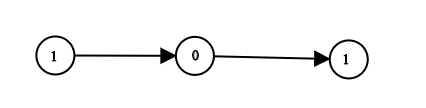LeetCode - LinkedList - Convert Binary Number in a Linked List to Integer

Given head which is a reference node to a singly-linked list. The value of each node in the linked list is either 0 or 1. The linked list holds the binary representation of a number.

Return the decimal value of the number in the linked list.

Example 1:Output: 5
Explanation: (101) in base 2 = (5) in base 10

/**
* public class ListNode {
*     int val;
*     ListNode next;
*     ListNode(int x) { val = x; }
* }
*/
class Solution {
String num = "";
while(temp != null){
num = num+temp.val;
temp = temp.next;
}
//System.out.println(num);
int decimalValue = Integer.parseInt(num, 2);
return decimalValue;
}
}